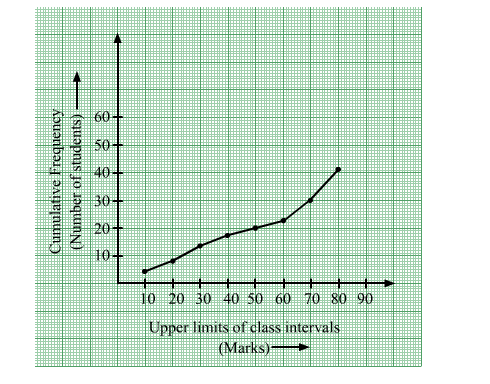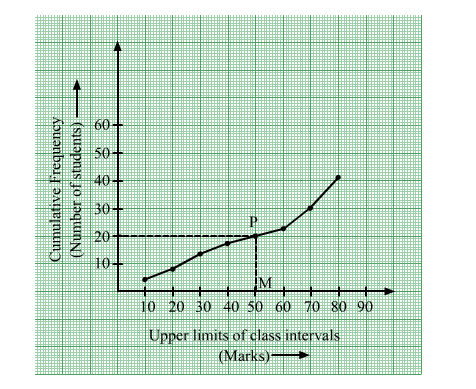# A student draws a cumulative frequency curve for the marks obtained

Question:

A student draws a cumulative frequency curve for the marks obtained by 40 students of a class as shown below. Find the median marks obtained by the students of the class.Solution:

Here, = 40

So, $\left(\frac{N}{2}\right)=20$

Draw a line parallel to x-axis from the point (0, 20), intersecting the graph at point P.Now, draw PM from P on the x-axis. The x-coordinate of M gives us the median.

$\therefore$ Median $=50$